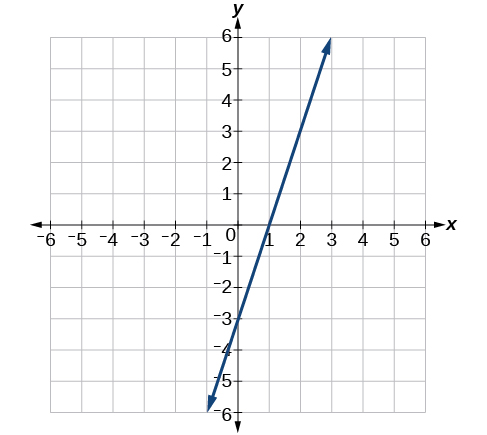$$\newcommand{\id}{\mathrm{id}}$$ $$\newcommand{\Span}{\mathrm{span}}$$ $$\newcommand{\kernel}{\mathrm{null}\,}$$ $$\newcommand{\range}{\mathrm{range}\,}$$ $$\newcommand{\RealPart}{\mathrm{Re}}$$ $$\newcommand{\ImaginaryPart}{\mathrm{Im}}$$ $$\newcommand{\Argument}{\mathrm{Arg}}$$ $$\newcommand{\norm}{\| #1 \|}$$ $$\newcommand{\inner}{\langle #1, #2 \rangle}$$ $$\newcommand{\Span}{\mathrm{span}}$$

2.R: Linear Functions(Review)

$$\newcommand{\vecs}{\overset { \rightharpoonup} {\mathbf{#1}} }$$ $$\newcommand{\vecd}{\overset{-\!-\!\rightharpoonup}{\vphantom{a}\smash {#1}}}$$$$\newcommand{\id}{\mathrm{id}}$$ $$\newcommand{\Span}{\mathrm{span}}$$ $$\newcommand{\kernel}{\mathrm{null}\,}$$ $$\newcommand{\range}{\mathrm{range}\,}$$ $$\newcommand{\RealPart}{\mathrm{Re}}$$ $$\newcommand{\ImaginaryPart}{\mathrm{Im}}$$ $$\newcommand{\Argument}{\mathrm{Arg}}$$ $$\newcommand{\norm}{\| #1 \|}$$ $$\newcommand{\inner}{\langle #1, #2 \rangle}$$ $$\newcommand{\Span}{\mathrm{span}}$$ $$\newcommand{\id}{\mathrm{id}}$$ $$\newcommand{\Span}{\mathrm{span}}$$ $$\newcommand{\kernel}{\mathrm{null}\,}$$ $$\newcommand{\range}{\mathrm{range}\,}$$ $$\newcommand{\RealPart}{\mathrm{Re}}$$ $$\newcommand{\ImaginaryPart}{\mathrm{Im}}$$ $$\newcommand{\Argument}{\mathrm{Arg}}$$ $$\newcommand{\norm}{\| #1 \|}$$ $$\newcommand{\inner}{\langle #1, #2 \rangle}$$ $$\newcommand{\Span}{\mathrm{span}}$$

2.1 Linear Functions

1) Determine whether the algebraic equation is linear: $$2 x+3 y=7$$

Yes

2) Determine whether the algebraic equation is linear: $$6 x^{2}-y=5$$

3) Determine whether the function is increasing or decreasing: $$f(x)=7 x-2$$

Increasing

4) Determine whether the function is increasing or decreasing: $$g(x)=-x+2$$

5) Given each set of information, find a linear equation that satisfies the given conditions, if possible. Passes through $$(7,5)$$ and $$(3,17)$$

$$y=-3 x+26$$

6) Given each set of information, find a linear equation that satisfies the given conditions, if possible. $$x$$ -intercept at $$(6,0)$$ and $$y$$ -intercept at $$(0,10)$$

7) Find the slope of the line shown in the line graph.$$3$$

8) Find the slope of the line graphed.9) Write an equation in slope-intercept form for the line shown.$$y=2 x-2$$

10) Does the following table represent a linear function? If so, find the linear equation that models the data.

 $$x$$ $$g(x)$$ –4 0 2 10 18 –2 –12 –52

11) Does the following table represent a linear function? If so, find the linear equation that models the data.

 $$x$$ $$g(x)$$ 6 8 12 26 –8 –12 –18 –46

Not linear

12) On June 1st, a company has $$\4,000,000$$ profit. If the company then loses $$150,000$$ dollars per day thereafter in the month of June, what is the company’s profit nth day after June 1st?

2.2 Graphs of Linear Functions

For the exercises 1-2, determine whether the lines given by the equations below are parallel, perpendicular, or neither parallel nor perpendicular:

1) \begin{align*} 2x-6y &=12 \\ -x+3y &=1 \end{align*} \nonumber

parallel

2) \begin{align*} y &=\dfrac{1}{3} x-2 \\ 3x+y &=-9 \end{align*} \nonumber

For the exercises 3-4, find the $$x$$- and $$y$$- intercepts of the given equation

3) $$7 x+9 y=-63$$

$$(-9,0); (0,-7)$$

4) $$f(x)=2 x-1$$

For the exercises 5-11, use the descriptions of the pairs of lines to find the slopes of Line 1 and Line 2. Is each pair of lines parallel, perpendicular, or neither?

5)

Line 1: Passes through $$(5,11)$$ and $$(10,1)$$
Line $$2 :$$ Passes through $$(-1,3)$$ and $$(-5,11)$$

Line 1 : $$m=-2$$; Line 2 : $$m=-2$$; Parallel

6)

Line 1: Passes through $$(8,-10)$$ and $$(0,-26)$$
Line 2: Passes through $$(2,5)$$ and $$(4,4)$$

7) Write an equation for a line perpendicular to $$f(x)=5 x-1$$ and passing through the point $$(5,20)$$.

$$y=-0.2 x+21$$

8) Find the equation of a line with a $$y$$ -intercept of $$(0,2)$$ and slope $$-\dfrac{1}{2}$$.

9) Sketch a graph of the linear function $$f(t)=2t-5$$.10) Find the point of intersection for the $$2$$ linear functions: \begin{align*} x &=y+6 \\ 2x-y &=13 \end{align*}

11) A car rental company offers two plans for renting a car.

Plan A: $$25$$ dollars per day and $$10$$ cents per mile

Plan B: $$50$$ dollars per day with free unlimited mileage

How many miles would you need to drive for plan B to save you money?

$$250$$

2.3 Modeling with Linear Functions

1) Find the area of a triangle bounded by the $$y$$ axis, the line $$f(x)=10-2x$$, and the line perpendicular to $$f$$ that passes through the origin.

2) A town’s population increases at a constant rate. In 2010 the population was $$55,000$$. By 2012 the population had increased to $$76,000$$. If this trend continues, predict the population in 2016.

$$118,000$$

3) The number of people afflicted with the common cold in the winter months dropped steadily by $$50$$ each year since 2004 until 2010. In 2004, $$875$$ people were inflicted.

4) Find the linear function that models the number of people afflicted with the common cold $$C$$ as a function of the year, $$t$$. When will no one be afflicted?

For the exercises 5-6, use the graph in the Figure below showing the profit, $$y$$, in thousands of dollars, of a company in a given year, $$x$$, where $$x$$ represents years since 1980.5) Find the linear function $$y$$, where $$y$$ depends on $$x$$, the number of years since 1980.

$$y=-300 x+11,500$$

6) Find and interpret the $$y$$-intercept.

7) For the following exercise, consider this scenario: In 2004, a school population was $$1,700$$. By 2012 the population had grown to $$2,500$$. Assume the population is changing linearly.

1. How much did the population grow between the year 2004 and 2012?
2. What is the average population growth per year?
3. Find an equation for the population, $$P$$, of the school $$t$$ years after 2004.
1. $$800$$
2. $$100$$ students per year
3. $$P(t)=100 t+1700$$
For the exercises 8-9, consider this scenario: In 2000, the moose population in a park was measured to be $$6,500$$. By 2010, the population was measured to be $$12,500$$. Assume the population continues to change linearly.